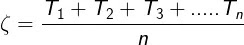# Is Drift Velocity Independent of time?

Electricity is an important part of our day to day life and we all know about the current and electricity. Current is created due to moving electron and electron moves due to drift velocity but the thing which makes me wonder is, is drift velocity independent of time ? and therefore I did some research on this.

Yes, drift velocity is independent of time as shown in the equation of drift velocity.

Let's see by deriving the equation of drift velocity and find how exactly is drift velocity independent of time ?

## Drift velocity independent of time:

So before learning about how drift velocity is independent of time we need to first define what drift velocity is:

### What is drift velocity ?

In a conductor, when an electric field is applied across a conductor, free-electron experiences a force in the direction of higher potential from a lower potential and while it keeps moving with the force due to thermal velocity it drifts in the direction of force and this is called as drift velocity.

This drift velocity is the reason due to which electron moves inside a conductor and current is produced.Current flowing inside a conductor due to drift velocity

Yes, drift velocity is independent of time. Drift velocity is the reason due to which current flows inside a conductor. In the derivation of the equation of drift velocity, we can see that acceleration "a" is constant and relaxation time "乁" is kept constant.

So current and drift velocity does not depend on time.

### Derivation of drift velocity equation:

Electrons that are present inside the conductors, moves in random different directions, which causes a collision between them.

Electric current is caused when all the electrons present inside a conductor moves in one particular direction but in the above scenarios, it's totally opposite of it which causes random collisions everwhere.

Now, there's no fixed time when these collisions happen inside a conductor, and therefore let's suppose the time between the collision of any two pair of the electron is T1, In the same way, let's take T2 for the second pair of electrons and this continues for all the pair of electron present inside a conductor.

Let's, for example, suppose the total number of pairs be 'n' so we will calculate an average from T1 to Tn and this average is named as Relaxation time which can be supposed as the time period between collision between two electrons.

Relaxation time: "乁" is the time period for electron elapsed between two consecutive collisions.Relaxation time formula

We know that: V= U + at

Where, V = final velocity, U = initial velocity, a = acceleration and t = time.

If initial velocity 'U' = 0 then, V = at

Now we know that acceleration:

We know that.

This average velocity is due to the application of E in one direction. Hence this is drift velocity.

In the equation of drift velocity 'Vd' depends upon the electric field 'E 'and Relaxation time '乁'.

Conclusion:

In the equation of drift velocity 'Vd' depends upon electric field and relaxation time. If temperature increases the relaxation time will decrease resulting in the decrease of drift velocity.

For a constant temperature relaxation time is constant so we can say that yes, drift velocity is independent of the time at a constant temperature.

#### F.A.Q:

Q.1 How drift velocity is responsible for the current ?

This drift velocity is responsible for current because this is not cancelled out. This drift velocity is very small but still, it is effective to make current because number of electron carrying in the same direction is extremely large.

Q.2 Why is acceleration constant here ?

The acceleration only depends on the applied electric field on a conductor (due to potential difference created by a battery).

Q.3 Why is the relaxation time also constant ?

The relaxation time is the average time. A collision of an electron is related to kinetic energy, therefore, it depends upon the temperature. So for a particular temperature relaxation time is kept constant.

Q.4 How does acceleration of an object and its dependency of time-related ?

The average value is used in the above equations. Initial velocity 'U' is zero because the motion of an electron in the absence of an electric field will be distributed in all the direction of conductor resulting in the net velocity to be zero. The equation used here is V= U+at

1.1.